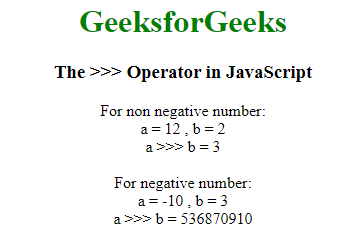GeeksforGeeks App
Open AppBrowser
Continue

# What is JavaScript >>> Operator and how to use it ?

The JavaScript >>> represents the zero-fill right shift operator. It is also called the unsigned right-bit shift operator. It comes under the category of Bitwise operators. Bitwise operators treat operands as 32-bit integer numbers and operate on their binary representation.

Zero-fill right shift (>>>) operator: It is a binary operator, where the first operand specifies the number and the second operand specifies the number of bits to shift. The operator shifts the bits of the first operand by a number of bits specified by the second operand. The bits are shifted to the right and those excess bits are discarded, while 0 bit is added from the left. As the sign bit becomes 0, the operator ( >>> ) returns a 32-bit non-negative integer.

Example:

```Input:
A = 6 ( 00000000000000000000000000000110 )
B = 1 ( 00000000000000000000000000000001 )

Output:
A >>> B = 3 ( 00000000000000000000000000000011 )```

Syntax:

`result = expression1 >>> expression2`

Difference between >>> and >>: The difference between these two is that the unsigned zero-fill right shift operator (>>>) fills with zeroes from the left, and the signed right bit shift operator (>>) fills with the sign bit from the left, thus it maintains the sign of the integer value when shifted.

Example: This example implements the use of >>> operator:

## html

 `<``body` `style``=``"text-align: center"``>``    ``<``h1` `style``=``"color: green"``>``        ``GeeksforGeeks``    ````    ``<``h3``>The >>> Operator in JavaScript`` ` `    ``<``script``>``        ``document.write("For non negative number:<``br``>");``        ``var a = 12;``         ` `        ``// Shift right two bits``        ``var b = 2;``        ``document.write("a = " + a + " , b = " + b);``        ``document.write("<``br``>a >>> b = " + (a >>> b) + '<``br``>');``         ` `        ``document.write("<``br``>For negative number:<``br``>");``        ``var a = -10;``             ` `        ``// Shift right two bits``        ``var b = 3;``        ``document.write("a = " + a + " , b = " + b);``        ``document.write("<``br``>a >>> b = " + (a >>> b) + '<``br``>');``    `````

Output:Explanation: For non-negative numbers, zero-fill right shift (>>>) and sign-propagating right shift (>>) gives the same output. For example, 9 >>> 2 and 9 >> 2 give the same result i.e. 2. But for negative numbers, -9 >>> 2 gives 1073741821, and -9 >> 2 gives -3 as output.

```Case 1: non-negative number
12 (base 10): 00000000000000000000000000001100 (base 2)
--------------------------------
12 >>> 2 (base 10): 00000000000000000000000000000011 (base 2)
= 3 (base 10)

Case 2: negative number
-10 (base 10): 11111111111111111111111111110110 (base 2)
--------------------------------
-10 >>> 3 (base 10): 00011111111111111111111111111110 (base 2)
= 536870910 (base 10) ```

My Personal Notes arrow_drop_up
Related Tutorials